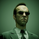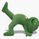7961 views
This implements the Even Better Sinewave indicator as described in the book Cycle Analysis for Traders by John F. Ehlers.
In the example I used 36 as the cycle to be analyzed and a second cycle with a shorter period, 9, the larger period tells where the dominant cycle is heading, and the faster cycle signals entry/exit points and reversals.
```// Madrid : 09/Jun/2015 21:09 : Even Better Sinewave : 1.0
// This implements the Even Better Sinewave indicator
// Ref. Cycle Analysis for Traders by John F. Ehlers.
//

Duration = input(36)
src = close

OB = 0.85, OS = -0.85
PI = 3.14159265358979

deg*PI/180.0

lowerBand = input(9)
ssFilter( price, lowerBand ) =>
angle = sqrt(2)*PI/lowerBand
a1= exp(-angle)
b1 = 2*a1*cos(angle)
c2 = b1
c3 = -a1*a1
c1 = 1 - c2 -c3
filt = c1*(price + nz(price))/2 + c2*nz(filt) + c3*nz(filt)

// HighPass filter cyclic components whose periods are shorter than Duration input
x = src
alpha1 = ( 1-sin(angle) ) / cos(angle)
HP = 0.5*(1+alpha1)*(x-x) + alpha1*nz(HP,0)

// Smooth with a Super Smoother Filter
Filt = ssFilter( HP, lowerBand )

Wave = ( Filt + nz(Filt,0) + nz(Filt,0) ) / 3
Pwr = ( Filt*Filt + nz(Filt,0)* nz(Filt,0) +  nz(Filt,0)* nz(Filt,0) ) /3

// Normalize the Average Wave to Square Root of the Average Power
sineWave = Wave / sqrt(Pwr)

// Output
sineWaveColor = sineWave>OB?green
:  sineWave<OS?red
:  change(sineWave)>0?green
:  red

plot( sineWave, color=sineWaveColor, linewidth=3 )
plot( sineWave, color=sineWaveColor, linewidth=1, style=histogram )

hline(0, color=silver, linestyle=dotted)

```

## コメントVery interesting
Do you have any example how using it? and di you use 2 different TF or lenght?
thxIncredible! I have recently become a big fan of John Ehlers. Been making money on binary options with indicators coded by the excellent programmers we have in TV community. Thanks.Wow...! This indicator work well for any TF.